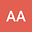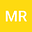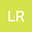loading page

Numerical study of flow across an ellipse and a circle placed in a uniform stream of infinite extent
•••Liudmila Rivkind
Institute of Applied Mathematics, TU Dortmund, Germany
Author Profile## Abstract

As an example of an aerodynamics prototypical study, we examined a two-dimensional low Reynolds number flow over obstacles immersed in a stream of infinite extent. The Navier Stokes equation is being discretized by non conforming finite element method approach. The resulting discretized nonlinear algebraic system is being solved by using the fixpoint method and the Newton method and multigrid method for the linear sub-problem employed. The magnitude of the uniform upstream velocity under the study of the problem for Reynolds number in the range 1 < Re < 100 and the angle of attack of the upstream velocity at α = -5; 0; 5 degrees performed. Analysis of the resulting drag and lift forces acting on obstacles with respect to the angle of attack of the upstream velocity and the Reynolds number is made. Moreover, the influence of one obstacle on the resulting drag and lift coefficients of other obstacles determined. The results are being presented in a graphical and vector form.